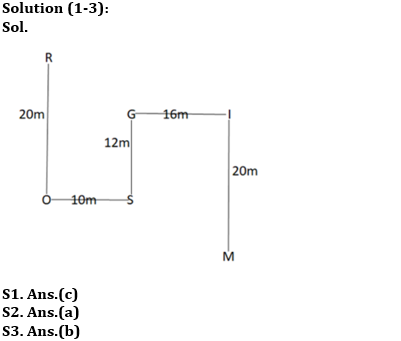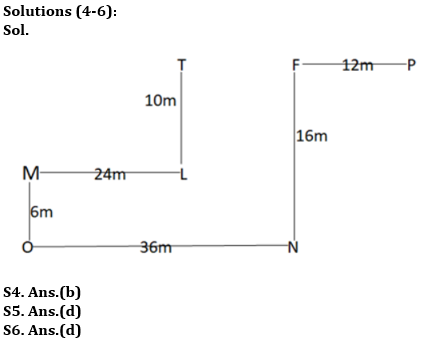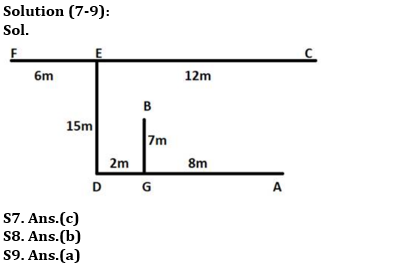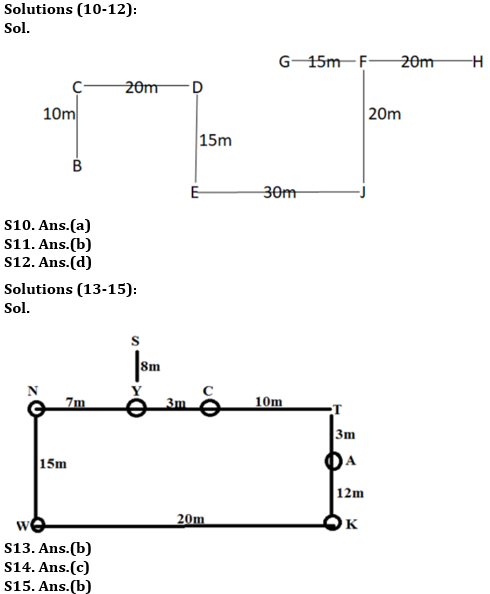Latest Banking jobs   »

# Reasoning Ability Quiz For RBI Grade B/ ECGC PO Pre 2022- 31st March

Directions (1-3): Study the data carefully and answer the questions accordingly.
Amar starts walking from point R and walks 20m south to reach point O. Now, he took a left turn and walks 10m to reach point S. Then, he took the left turn and he walks 12m to reach point G. From here, he took a right turn and walks 16m to reach point I. Finally, he turned south and walks 20m to reach point M.

Q1. What is the total distance between S and M?
(a) 52m
(b) 43m
(c) 48m
(d) 54m
(e) None of these

Q2. Point O is in which direction of point G?
(a) South-West
(b) South-East
(c) West
(d) North-East
(e) None of these

Q3. Point I is in which direction of point R?
(a) North-West
(b) South-East
(c) East
(d) North-East
(e) None of these

Directions (4-6): Study the data carefully and answer the questions accordingly.
Point F is north of point N. Point P is 12m east of point F. Point L is 10m south of point T. Point M is 24m west of point L. Point O is 6m south of point M. Point N is 36m east of point O. Point F and T are in the same line.

Q4. What is the distance between T and P?
(a) 28m
(b) 24m
(c) 31m
(d) 30m
(e) None of these

Q5. Point L is in which direction of point F?
(a) North
(b) South
(c) North-East
(d) South-West
(e) None of these

Q6. Find the odd one.
(a) P, N
(b) T, M
(c) P, L
(d) N, M
(e) F, O

Directions (7-9): Read the following information carefully and answer the questions which follow.

Seven friends A, B, C, D, E, F, and G are standing on the ground. A is 8m to the east of G. D is 10m to the west of A. E is 15m to the north of D. F is 6m to the west of E . C is 12m to the east of E. B is 7m to the north of G.

Q7. What is the direction of B with respect to F?
(a) South
(b) South-west
(c) South-east
(d) North-west
(e) None of these

Q8. If N is 8m to the south of F, then what is the shortest distance between N and E?
(a) 12m
(b) 10m
(c) 8m
(d) 5m
(e) None of these

Q9. What is the direction of G with respect to E and C respectively?
(a) South-east and South-west
(b) East and South-east
(c) West and South
(d) North-west and North-east
(e) None of these

Directions (10-12): Study the data carefully and answer the questions accordingly.
Point E is 30m west of point J. Point H is 20m east of point F. Point C is 10m north of point B. Point D is 15m north of point E and 20m east of point C. Point F is 20m north of point J. Point G is 15m west of point F.

Q10. Point E is in which direction of point H?
(a) South-West
(b) South
(c) North-East
(d) East
(e) None of these

Q11. What is the shortest distance between the points G and C?
(a) 30m
(b) 25√2m
(c) 35m
(d) 35√2m
(e) None of these

Q12. Which of the following points are in a straight line?
(a) C, D, G
(b) F, H, D
(c) F, J, G
(d) G, F, H
(e) None of these

Directions (13-15): Study the data carefully and answer the questions accordingly.
Point N is 7m to the west of Point Y, which is 8m to the south of Point S. Point N is 15m to the north of Point W and 10m to the west of Point C. Point A is 12m to the north of Point K which is 20m to the east of Point W. A is to the south of T which forms a straight line with Y.

Q13. N is at what distance and in which direction with respect to K?
(a) 24m, North-West
(b) 25m, North-West
(c) 25m, North-East
(d) 24m, East
(e) 25m, North

Q14. If a person starts walking from N to K via Y, C, T, and A. How many meters will he walk?
(a) 40m
(b) 45m
(c) 35m
(d) 20m
(e) 25m

Q15. S is at which direction from point K?
(a) North East
(b) North West
(c) South East
(d) South West
(e) None of these

Solutions#### Congratulations!